# Precalculus : Solve Exponential Growth Problems

## Example Questions

### Example Question #1 : Growth And Decay

Stuff animals were a strange craze of the 90's. A Cat stuff animal with white paws sold for $6 in 1997. In 2015, the Cat will sell for$1015. What has been the approximate rate of growth for these stuff animal felines?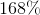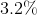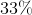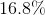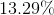Explanation:

Use the formula for exponential growth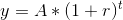where y is the current value, A is the initial value, r is the rate of growth, and t is time. Between 1997 and 2015, 18 years passed, so use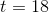. The stuffed animal was originally worth $6, so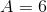. It is now worth$1,015, so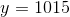.

Our equation is now: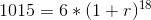divide by 6: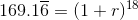take both sides to the power of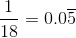: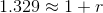subtract 1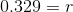As a percent, r is about 33%.

### All Precalculus Resources